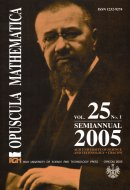Opuscula Math. 25, no. 1 (2005), 131-137

Opuscula Mathematica

# Recovering a part of potential by partial information on spectra of boundary problems

Vyacheslav Pivovarchik

Abstract. Under additional conditions uniqueness of the solution is proved for the following problem. Given 1) the spectrum of the Dirichlet problem for the Sturm-Liouville equation on $$[0,a]$$ with real potential $$q(x)\in L_2(0,a)$$, 2) a certain part of the spectrum of the Dirichlet problem for the same equation on $$[\frac{a}{3},a]$$ and 3) the potential on $$[0,\frac{a}{3}]$$. The aim is to find the potential on $$[\frac{a}{3},a]$$.

Keywords: sine-type function, Lagrange interpolation series, Dirichlet boundary value problem, Dirichlet-Neumann boundary value problem.

Mathematics Subject Classification: 34B24, 34A55, 34B10, 73K03.

Full text (pdf)

• Vyacheslav Pivovarchik
• South-Ukrainian State Pedagogical University, Department of Applied Mathematics and Informatics, Staropotofrankovskaya 26, Odessa, Ukraine 65029